xHubble's lawEncyclopedia
Hubble's law is the name for the astronomical observation in physical cosmology
Physical cosmology
Physical cosmology, as a branch of astronomy, is the study of the largest-scale structures and dynamics of the universe and is concerned with fundamental questions about its formation and evolution. For most of human history, it was a branch of metaphysics and religion...

that: (1) all objects observed in deep space (interstellar space) are found to have a doppler shift observable relative velocity to Earth, and to each other; and (2) that this doppler-shift-measured velocity, of various galaxies
Galaxy
A galaxy is a massive, gravitationally bound system that consists of stars and stellar remnants, an interstellar medium of gas and dust, and an important but poorly understood component tentatively dubbed dark matter. The word galaxy is derived from the Greek galaxias , literally "milky", a...

receding from the Earth, is proportional
Proportionality (mathematics)
In mathematics, two variable quantities are proportional if one of them is always the product of the other and a constant quantity, called the coefficient of proportionality or proportionality constant. In other words, are proportional if the ratio \tfrac yx is constant. We also say that one...

to their distance from the Earth and all other interstellar bodies. In effect, the space-time volume of the observable universe is expanding and Hubble's law is the direct physical observation of this process. It is considered the first observational basis for the expanding space paradigm
Metric expansion of space
The metric expansion of space is the increase of distance between distant parts of the universe with time. It is an intrinsic expansion—that is, it is defined by the relative separation of parts of the universe and not by motion "outward" into preexisting space...

and today serves as one of the pieces of evidence most often cited in support of the Big Bang
Big Bang
The Big Bang theory is the prevailing cosmological model that explains the early development of the Universe. According to the Big Bang theory, the Universe was once in an extremely hot and dense state which expanded rapidly. This rapid expansion caused the young Universe to cool and resulted in...

model.

Although widely attributed to Edwin Hubble
Edwin Hubble
Edwin Powell Hubble was an American astronomer who profoundly changed the understanding of the universe by confirming the existence of galaxies other than the Milky Way - our own galaxy...

, the law was first derived from the General Relativity
General relativity
General relativity or the general theory of relativity is the geometric theory of gravitation published by Albert Einstein in 1916. It is the current description of gravitation in modern physics...

equations by Georges Lemaître
Georges Lemaître
Monsignor Georges Henri Joseph Édouard Lemaître was a Belgian priest, astronomer and professor of physics at the Catholic University of Louvain. He was the first person to propose the theory of the expansion of the Universe, widely misattributed to Edwin Hubble...

in a 1927 article where he proposed that the Universe is expanding
Metric expansion of space
The metric expansion of space is the increase of distance between distant parts of the universe with time. It is an intrinsic expansion—that is, it is defined by the relative separation of parts of the universe and not by motion "outward" into preexisting space...

and suggested an estimated value of the rate of expansion, now called the Hubble constant. 2 years later Edwin Hubble
Edwin Hubble
Edwin Powell Hubble was an American astronomer who profoundly changed the understanding of the universe by confirming the existence of galaxies other than the Milky Way - our own galaxy...

confirmed the existence of that law and determined a more accurate value for the constant that now bears his name. The recession velocity of the objects was inferred from their redshifts, many measured earlier by Vesto Slipher
Vesto Slipher
Vesto Melvin Slipher was an American astronomer. His brother Earl C. Slipher was also an astronomer and a director at the Lowell Observatory....

(1917) and related to velocity by him.

The law is often expressed by the equation , with H0 the constant of proportionality (the Hubble constant) between the "proper distance" D to a galaxy (which can change over time, unlike the comoving distance
Comoving distance
In standard cosmology, comoving distance and proper distance are two closely related distance measures used by cosmologists to define distances between objects...

) and its velocity v (i.e. the derivative
Derivative
In calculus, a branch of mathematics, the derivative is a measure of how a function changes as its input changes. Loosely speaking, a derivative can be thought of as how much one quantity is changing in response to changes in some other quantity; for example, the derivative of the position of a...

of proper distance with respect to cosmological time coordinate; see Uses of the proper distance for some discussion of the subtleties of this definition of 'velocity'). The SI unit of H0 is s−1 but it is most frequently quoted in (km
Kilometre
The kilometre is a unit of length in the metric system, equal to one thousand metres and is therefore exactly equal to the distance travelled by light in free space in of a second...

/s
Second
The second is a unit of measurement of time, and is the International System of Units base unit of time. It may be measured using a clock....

)/Mpc, thus giving the speed in km/s of a galaxy 1 Mpc away. The reciprocal of H0 is the Hubble time.

A recent 2011 estimate of the Hubble constant, which used a new infrared camera on the Hubble Space Telescope
Hubble Space Telescope
The Hubble Space Telescope is a space telescope that was carried into orbit by a Space Shuttle in 1990 and remains in operation. A 2.4 meter aperture telescope in low Earth orbit, Hubble's four main instruments observe in the near ultraviolet, visible, and near infrared...

(HST) to measure the distance and redshift for a collection of astronomical objects, gives a value of . An alternate approach using data from galactic clusters gave a value of .

An observational determination of the Hubble constant obtained in 2010 based on measurements of gravitational lensing by using the HST yielded a value of . WMAP seven-year results, also from 2010, gave an estimate of based on WMAP data alone, and an estimate of based on WMAP data with Gaussian prior
Prior probability
In Bayesian statistical inference, a prior probability distribution, often called simply the prior, of an uncertain quantity p is the probability distribution that would express one's uncertainty about p before the "data"...

s based on earlier estimates from other studies. In 2009 also using the Hubble Space Telescope the measure was 74.2 ± 3.6 (km/s)/Mpc.
The results agree closely with an earlier measurement, based on observations by the HST of Cepheid variable
Cepheid variable
A Cepheid is a member of a class of very luminous variable stars. The strong direct relationship between a Cepheid variable's luminosity and pulsation period, secures for Cepheids their status as important standard candles for establishing the Galactic and extragalactic distance scales.Cepheid...

stars, of obtained in 2001. In August 2006, a less-precise figure was obtained independently using data from NASA's Chandra X-ray Observatory
Chandra X-ray Observatory
The Chandra X-ray Observatory is a satellite launched on STS-93 by NASA on July 23, 1999. It was named in honor of Indian-American physicist Subrahmanyan Chandrasekhar who is known for determining the maximum mass for white dwarfs. "Chandra" also means "moon" or "luminous" in Sanskrit.Chandra...

: or about 2.5×10−18 s−1 with an uncertainty of ± 15%. NASA's WMAP site summarizes existing data to indicate a constant of 70.8 ± 1.6 (km/s)/Mpc if space is assumed to be flat, or 70.8 ± 4.0 (km/s)/Mpc otherwise, although these estimates have been on the site since January 2007 and may not take into account the more recent studies discussed above.

## Discovery

A decade before Hubble made his observations, a number of physicists and mathematicians had established a consistent theory of the relationship between space and time
Spacetime
In physics, spacetime is any mathematical model that combines space and time into a single continuum. Spacetime is usually interpreted with space as being three-dimensional and time playing the role of a fourth dimension that is of a different sort from the spatial dimensions...

by using Einstein's field equations of general relativity
General relativity
General relativity or the general theory of relativity is the geometric theory of gravitation published by Albert Einstein in 1916. It is the current description of gravitation in modern physics...

. Applying the most general principles to the nature of the universe
Universe
The Universe is commonly defined as the totality of everything that exists, including all matter and energy, the planets, stars, galaxies, and the contents of intergalactic space. Definitions and usage vary and similar terms include the cosmos, the world and nature...

yielded a dynamic
Dynamics (mechanics)
In the field of physics, the study of the causes of motion and changes in motion is dynamics. In other words the study of forces and why objects are in motion. Dynamics includes the study of the effect of torques on motion...

solution that conflicted with the then prevailing notion of a static universe
Static universe
A static universe, also referred to as a "stationary" or "Einstein" universe, is a model in which space is neither expanding nor contracting. Albert Einstein proposed such a model as his preferred cosmology in 1917...

.

### FLRW equations

In 1922, Alexander Friedmann derived his Friedmann equations
Friedmann equations
The Friedmann equations are a set of equations in physical cosmology that govern the expansion of space in homogeneous and isotropic models of the universe within the context of general relativity...

from Einstein's field equations
Einstein field equations
The Einstein field equations or Einstein's equations are a set of ten equations in Albert Einstein's general theory of relativity which describe the fundamental interaction of gravitation as a result of spacetime being curved by matter and energy...

, showing that the universe might expand at a rate calculable by the equations. The parameter used by Friedmann is known today as the scale factor
Scale factor (Universe)
The scale factor or cosmic scale factor parameter of the Friedmann equations is a function of time which represents the relative expansion of the universe. It is sometimes called the Robertson-Walker scale factor...

which can be considered as a scale invariant form of the proportionality constant of Hubble's law. Georges Lemaître
Georges Lemaître
Monsignor Georges Henri Joseph Édouard Lemaître was a Belgian priest, astronomer and professor of physics at the Catholic University of Louvain. He was the first person to propose the theory of the expansion of the Universe, widely misattributed to Edwin Hubble...

independently found a similar solution in 1927. The Friedmann equations are derived by inserting the metric for a homogeneous and isotropic universe into Einstein's field equations for a fluid with a given density
Density
The mass density or density of a material is defined as its mass per unit volume. The symbol most often used for density is ρ . In some cases , density is also defined as its weight per unit volume; although, this quantity is more properly called specific weight...

and pressure
Pressure
Pressure is the force per unit area applied in a direction perpendicular to the surface of an object. Gauge pressure is the pressure relative to the local atmospheric or ambient pressure.- Definition :...

. This idea of an expanding spacetime would eventually lead to the Big Bang
Big Bang
The Big Bang theory is the prevailing cosmological model that explains the early development of the Universe. According to the Big Bang theory, the Universe was once in an extremely hot and dense state which expanded rapidly. This rapid expansion caused the young Universe to cool and resulted in...

In cosmology, the Steady State theory is a model developed in 1948 by Fred Hoyle, Thomas Gold, Hermann Bondi and others as an alternative to the Big Bang theory...

theories of cosmology.

### Shape of the universe

Before the advent of modern cosmology
Big Bang
The Big Bang theory is the prevailing cosmological model that explains the early development of the Universe. According to the Big Bang theory, the Universe was once in an extremely hot and dense state which expanded rapidly. This rapid expansion caused the young Universe to cool and resulted in...

, there was considerable talk about the size and shape
Shape of the Universe
The shape of the universe is a matter of debate in physical cosmology over the local and global geometry of the universe which considers both curvature and topology, though, strictly speaking, it goes beyond both...

of the universe
Universe
The Universe is commonly defined as the totality of everything that exists, including all matter and energy, the planets, stars, galaxies, and the contents of intergalactic space. Definitions and usage vary and similar terms include the cosmos, the world and nature...

. In 1920, the famous Shapley-Curtis debate took place between Harlow Shapley
Harlow Shapley
Harlow Shapley was an American astronomer.-Career:He was born on a farm in Nashville, Missouri, and dropped out of school with only the equivalent of a fifth-grade education...

and Heber D. Curtis
Heber Doust Curtis
Heber Doust Curtis was an American astronomer.He studied at the University of Michigan and at the University of Virginia, where he got a degree in astronomy....

over this issue. Shapley argued for a small universe the size of the Milky Way galaxy and Curtis argued that the universe was much larger. The issue was resolved in the coming decade with Hubble's improved observations.

### Cepheid variable stars outside of the Milky WayEdwin Hubble did most of his professional astronomical observing work at Mount Wilson Observatory
Mount Wilson Observatory
The Mount Wilson Observatory is an astronomical observatory in Los Angeles County, California, United States. The MWO is located on Mount Wilson, a 5,715 foot peak in the San Gabriel Mountains near Pasadena, northeast of Los Angeles...

, the world's most powerful telescope at the time. His observations of Cepheid variable
Cepheid variable
A Cepheid is a member of a class of very luminous variable stars. The strong direct relationship between a Cepheid variable's luminosity and pulsation period, secures for Cepheids their status as important standard candles for establishing the Galactic and extragalactic distance scales.Cepheid...

stars in spiral nebulae enabled him to calculate the distances to these objects. Surprisingly, these objects were discovered to be at distances which placed them well outside the Milky Way
Milky Way
The Milky Way is the galaxy that contains the Solar System. This name derives from its appearance as a dim un-resolved "milky" glowing band arching across the night sky...

. They continued to be called "nebulae" and it was only gradually that the term "galaxies" took over.

### Combining redshifts with distance measurementsThe parameters that appear in Hubble’s law: velocities and distances, are not directly measured. In reality we determine, say, a supernova brightness, which provides information about its distance, and the redshift z = ∆λ/λ of its spectrum of radiation. Hubble correlated brightness and parameter z.

Combining his measurements of galaxy distances with Vesto Slipher
Vesto Slipher
Vesto Melvin Slipher was an American astronomer. His brother Earl C. Slipher was also an astronomer and a director at the Lowell Observatory....

and Milton Humason's measurements of the redshifts associated with the galaxies, Hubble discovered a rough proportionality between redshift of an object and its distance. Though there was considerable scatter
Variance
In probability theory and statistics, the variance is a measure of how far a set of numbers is spread out. It is one of several descriptors of a probability distribution, describing how far the numbers lie from the mean . In particular, the variance is one of the moments of a distribution...

(now known to be caused by peculiar velocities
Peculiar velocity
Peculiar motion or peculiar velocity refers to the true velocity of an object, relative to a rest frame.-Galactic astronomy:In galactic astronomy, the term peculiar motion refers to the motion of an object through space.Local objects are usually related in terms of proper motion and radial...

- the 'Hubble flow' is used to refer to the region of space far enough out that the recession velocity is larger than local peculiar velocities), Hubble was able to plot a trend line from the 46 galaxies he studied and obtain a value for the Hubble constant of 500 km/s/Mpc (much higher than the currently accepted value due to errors in his distance calibrations). (See cosmic distance ladder
The cosmic distance ladder is the succession of methods by which astronomers determine the distances to celestial objects. A real direct distance measurement of an astronomical object is possible only for those objects that are "close enough" to Earth...

for details.)

At the time of discovery and development of Hubble’s law it was acceptable to explain redshift phenomenon as a Doppler shift in the context of special relativity, and use the Doppler formula to associate redshift z with velocity. Today the velocity-distance relationship of Hubble's law is viewed as a theoretical result with velocity to be connected with observed redshift not by the Doppler effect, but by a cosmological model relating recessional velocity to the expansion of the universe. Even for small z the velocity entering the Hubble law is no longer interpreted as a Doppler effect, although at small z the velocity-redshift relation for both interpretations is the same.

In 1958, the first good estimate of H0, 75 km/s/Mpc, was published by Allan Sandage
Allan Sandage
Allan Rex Sandage was an American astronomer. He was Staff Member Emeritus with the Carnegie Observatories in Pasadena, California. He is best known for determining the first reasonably accurate value for the Hubble constant and the age of the universe.-Career:Sandage was one of the most...

, but it would be decades before a consensus was achieved.

#### Hubble Diagram

Hubble's law can be easily depicted in a "Hubble Diagram" in which the velocity (assumed approximately proportional to the redshift) of an object is plotted with respect to its distance from the observer. A straight line of positive slope on this diagram is the visual depiction of Hubble's law.

### The cosmological constant abandoned

After Hubble's discovery was published, Albert Einstein
Albert Einstein
Albert Einstein was a German-born theoretical physicist who developed the theory of general relativity, effecting a revolution in physics. For this achievement, Einstein is often regarded as the father of modern physics and one of the most prolific intellects in human history...

abandoned his work on the cosmological constant
Cosmological constant
In physical cosmology, the cosmological constant was proposed by Albert Einstein as a modification of his original theory of general relativity to achieve a stationary universe...

, which he had designed to modify his equations of general relativity, to allow them to produce a static solution which, as originally formulated, his equations did not admit. He later termed this work his "greatest blunder" since it was his incorrect presumption of a static universe that had caused him to fail to accept what could be seen in his concepts and equations of general relativity: the fact that general relativity was both predicting and providing the means for calculating the expansion of the universe, which (like the bending of light by large masses or the precession of the orbit of Mercury) could be experimentally observed and compared to his theoretical calculations using particular solutions of the equations of general relativity as he had originally formulated them.

Einstein made a famous trip to Mount Wilson in 1931 to thank Hubble for providing the observational basis for modern cosmology.

The cosmological constant has regained attention in recent decades as a hypothesis for dark energy
Dark energy
In physical cosmology, astronomy and celestial mechanics, dark energy is a hypothetical form of energy that permeates all of space and tends to accelerate the expansion of the universe. Dark energy is the most accepted theory to explain recent observations that the universe appears to be expanding...

.

## Interpretation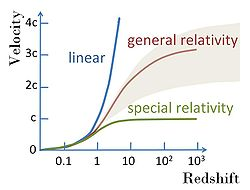The discovery of the linear relationship between redshift
Redshift
In physics , redshift happens when light seen coming from an object is proportionally increased in wavelength, or shifted to the red end of the spectrum...

and distance, coupled with a supposed linear relation between recessional velocity
Recessional velocity
Recessional Velocity is a term used to describe the rate at which an object is moving away, typically from Earth.-Application to Cosmology:This term is generally only used in reference to distant Galaxies. The most common reason for the use of this term is Hubble's Law, which states that the...

and redshift, yields a straightforward mathematical expression for Hubble's Law as follows: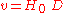where
•is the recessional velocity, typically expressed in km/s.
• H0 is Hubble's constant and corresponds to the value of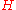(often termed the Hubble parameter which is a value that is time dependent
Time-variant system
A time-variant system is a system that is not time invariant . Roughly speaking, characteristics of its output depend explicitly upon time.- Overview :...

and which can be expressed in terms of the scale factor) in the Friedmann equations taken at the time of observation denoted by the subscript 0. This value is the same throughout the universe for a given comoving time.
•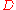is the proper distance (which can change over time, unlike the comoving distance
Comoving distance
In standard cosmology, comoving distance and proper distance are two closely related distance measures used by cosmologists to define distances between objects...

which is constant) from the galaxy
Galaxy
A galaxy is a massive, gravitationally bound system that consists of stars and stellar remnants, an interstellar medium of gas and dust, and an important but poorly understood component tentatively dubbed dark matter. The word galaxy is derived from the Greek galaxias , literally "milky", a...

to the observer, measured in mega parsec
Parsec
The parsec is a unit of length used in astronomy. It is about 3.26 light-years, or just under 31 trillion kilometres ....

s (Mpc), in the 3-space defined by given cosmological time. (Recession velocity is just v = dD/dt).

Hubble's law is considered a fundamental relation between recessional velocity and distance. However, the relation between recessional velocity and redshift depends on the cosmological model adopted, and is not established except for small redshifts.

For distances D larger than the radius of the Hubble sphere rHS , objects recede at a rate faster than the speed of light
Speed of light
The speed of light in vacuum, usually denoted by c, is a physical constant important in many areas of physics. Its value is 299,792,458 metres per second, a figure that is exact since the length of the metre is defined from this constant and the international standard for time...

(See Uses of the proper distance for a discussion of the significance of this):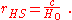Since the Hubble "constant" is only a constant in space, not in time, the radius of the Hubble sphere may increase or decrease over various time intervals. The subscript '0' indicates the value of the Hubble constant today. Current evidence suggests the expansion of the universe is accelerating (see Accelerating universe
Accelerating universe
The accelerating universe is the observation that the universe appears to be expanding at an increasing rate, which in formal terms means that the cosmic scale factor a has a positive second derivative, implying that the velocity at which a given galaxy is receding from us should be continually...

), meaning that for any given galaxy, the recession velocity dD/dt is increasing over time as the galaxy moves to greater and greater distances; however, the Hubble parameter is actually thought to be decreasing with time, meaning that if we were to look at some fixed distance D and watch a series of different galaxies pass that distance, later galaxies would pass that distance at a smaller velocity than earlier ones.

### Redshift velocity and recessional velocity

Redshift
Redshift
In physics , redshift happens when light seen coming from an object is proportionally increased in wavelength, or shifted to the red end of the spectrum...

can be measured by determining the wavelength of a known transition, such as hydrogen α-lines for distant quasars, and finding the fractional shift compared to a stationary reference. Thus redshift is a quantity unambiguous for experimental observation. The relation of redshift to recessional velocity is another matter. For an extensive discussion, see Harrison.

#### Redshift velocity

The redshift z often is described as a redshift velocity, which is the recessional velocity that would produce the same redshift if it were caused by a linear Doppler effect
Doppler effect
The Doppler effect , named after Austrian physicist Christian Doppler who proposed it in 1842 in Prague, is the change in frequency of a wave for an observer moving relative to the source of the wave. It is commonly heard when a vehicle sounding a siren or horn approaches, passes, and recedes from...

(which, however, is not the case, as the shift is caused in part by a cosmological expansion of space
Metric expansion of space
The metric expansion of space is the increase of distance between distant parts of the universe with time. It is an intrinsic expansion—that is, it is defined by the relative separation of parts of the universe and not by motion "outward" into preexisting space...

, and because the velocities involved are too large to use a non-relativistic formula for Doppler shift). This redshift velocity can easily exceed the speed of light. In other words, to determine the redshift velocity vrs, the relation: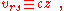is used. That is, there is no fundamental difference between redshift velocity and redshift: they are rigidly proportional, and not related by any theoretical reasoning. The motivation behind the "redshift velocity" terminology is that the redshift velocity agrees with the velocity from a low-velocity simplification of the so-called Fizeau-Doppler formula
Relativistic Doppler effect
The relativistic Doppler effect is the change in frequency of light, caused by the relative motion of the source and the observer , when taking into account effects described by the special theory of relativity.The relativistic Doppler effect is different from the non-relativistic Doppler effect...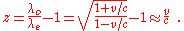Here, λo, λe are the observed and emitted wavelengths respectively. The "redshift velocity" vrs is not so simply related to real velocity at larger velocities, however, and this terminology leads to confusion if interpreted as a real velocity. Next, the connection between redshift or redshift velocity and recessional velocity is discussed. This discussion is based on Sartori.

#### Recessional velocity

Suppose R(t) is called the scale factor
Scale factor (Universe)
The scale factor or cosmic scale factor parameter of the Friedmann equations is a function of time which represents the relative expansion of the universe. It is sometimes called the Robertson-Walker scale factor...

of the universe, and increases as the universe expands in a manner that depends upon the cosmological model selected. Its meaning is that all measured distances D(t) between co-moving points increase proportionally to R. (The co-moving points are not moving relative to each other except as a result of the expansion of space.) In other words: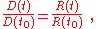where t0 is some reference time. If light is emitted from a galaxy at time te and received by us at t0, it is red shifted due to the expansion of space, and this redshift z is simply: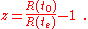Suppose a galaxy is at distance D, and this distance changes with time at a rate dtD . We call this rate of recession the "recession velocity" vr: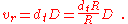We now define the Hubble constant as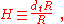and discover the Hubble law: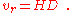From this perspective, Hubble's law is a fundamental relation between (i) the recessional velocity contributed by the expansion of space and (ii) the distance to an object; the connection between redshift and distance is a crutch used to connect Hubble's law with observations. This law can be related to redshift z approximately by making a Taylor series
Taylor series
In mathematics, a Taylor series is a representation of a function as an infinite sum of terms that are calculated from the values of the function's derivatives at a single point....

expansion: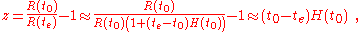If the distance is not too large, all other complications of the model become small corrections and the time interval is simply the distance divided by the speed of light: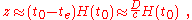or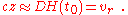According to this approach, the relation cz = vr is an approximation valid at low redshifts, to be replaced by a relation at large redshifts that is model-dependent. See velocity-redshift figure.

### Observability of parameters

Strictly speaking, neither v nor D in the formula are directly observable, because they are properties now of a galaxy, whereas our observations refer to the galaxy in the past, at the time that the light we currently see left it.

For relatively nearby galaxies (redshift
Redshift
In physics , redshift happens when light seen coming from an object is proportionally increased in wavelength, or shifted to the red end of the spectrum...

z much less than unity), v and D will not have changed much, and v can be estimated using the formulawhere c is the speed of light
Speed of light
The speed of light in vacuum, usually denoted by c, is a physical constant important in many areas of physics. Its value is 299,792,458 metres per second, a figure that is exact since the length of the metre is defined from this constant and the international standard for time...

. This gives the empirical relation found by Hubble.

For distant galaxies, v (or D) cannot be calculated from z without specifying a detailed model for how H changes with time. The redshift is not even directly related to the recession velocity at the time the light set out, but it does have a simple interpretation: (1+z) is the factor by which the universe has expanded while the photon was travelling towards the observer.

### Expansion velocity vs relative velocity

In using Hubble's law to determine distances, only the velocity due to the expansion of the universe can be used. Since gravitationally interacting galaxies move relative to each other independent of the expansion of the universe, these relative velocities, called peculiar velocities, need to be accounted for in the application of Hubble's law.

The Finger of God
Fingers of God
Fingers of God is an effect in observational cosmology that causes clusters of galaxies to be elongated in redshift space, with an axis of elongation pointed toward the observer. It is caused by a Doppler shift associated with the peculiar velocities of galaxies in a cluster...

effect, discovered in 1938 by Benjamin Kenneally, is one result of this phenomenon. In systems that are gravitationally bound, such as galaxies or our planetary system, the expansion of space is a much weaker effect than the attractive force of gravity.

### Idealized Hubble's Law

The mathematical derivation of an idealized Hubble's Law for a uniformly expanding universe is a fairly elementary theorem of geometry in 3-dimensional Cartesian
Cartesian coordinate system
A Cartesian coordinate system specifies each point uniquely in a plane by a pair of numerical coordinates, which are the signed distances from the point to two fixed perpendicular directed lines, measured in the same unit of length...

/Newtonian coordinate space, which, considered as a metric space
Metric space
In mathematics, a metric space is a set where a notion of distance between elements of the set is defined.The metric space which most closely corresponds to our intuitive understanding of space is the 3-dimensional Euclidean space...

, is entirely homogeneous and isotropic
Cosmological Principle
In modern physical cosmology, the cosmological principle is the working assumption that observers on Earth do not occupy an unusual or privileged location within the universe as a whole, judged as observers of the physical phenomena produced by uniform and universal laws of physics...

(properties do not vary with location or direction). Simply stated the theorem is this:
Any two points which are moving away from the origin, each along straight lines and with speed proportional to distance from the origin, will be moving away from each other with a speed proportional to their distance apart.

In fact this applies to non-Cartesian spaces as long as they are locally homogeneous and isotropic; specifically to the negatively- and positively-curved spaces frequently considered as cosmological models (see shape of the universe
Shape of the Universe
The shape of the universe is a matter of debate in physical cosmology over the local and global geometry of the universe which considers both curvature and topology, though, strictly speaking, it goes beyond both...

).

An observation stemming from this theorem is that seeing objects recede from us on Earth is not an indication that Earth is near to a center from which the expansion is occurring, but rather that every observer in an expanding universe will see objects receding from them.

### The ‘ultimate fate’ and age of the universe

The value of the Hubble parameter changes over time either increasing or decreasing depending on the sign of the so-called deceleration parameter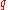which is defined by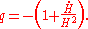In a universe with a deceleration parameter equal to zero, it follows that H = 1/t, where t is the time since the Big Bang. A non-zero, time-dependent value ofsimply requires integration
Integral
Integration is an important concept in mathematics and, together with its inverse, differentiation, is one of the two main operations in calculus...

of the Friedmann equations backwards from the present time to the time when the comoving horizon size was zero.

It was long thought that q was positive, indicating that the expansion is slowing down due to gravitational attraction. This would imply an age of the universe less than 1/H (which is about 14 billion years). For instance, a value for q of 1/2 (once favoured by most theorists) would give the age of the universe as 2/(3H). The discovery in 1998 that q is apparently negative means that the universe could actually be older than 1/H. However, estimates of the age of the universe
Age of the universe
The age of the universe is the time elapsed since the Big Bang posited by the most widely accepted scientific model of cosmology. The best current estimate of the age of the universe is 13.75 ± 0.13 billion years within the Lambda-CDM concordance model...

are very close to 1/H.

The expansion of space summarized by the Big Bang interpretation of Hubble's Law is relevant to the old conundrum known as Olbers' paradox
In astrophysics and physical cosmology, Olbers' paradox is the argument that the darkness of the night sky conflicts with the assumption of an infinite and eternal static universe. It is one of the pieces of evidence for a non-static universe such as the current Big Bang model. The argument is also...

: if the universe were infinite, static
Static universe
A static universe, also referred to as a "stationary" or "Einstein" universe, is a model in which space is neither expanding nor contracting. Albert Einstein proposed such a model as his preferred cosmology in 1917...

, and filled with a uniform distribution of star
Star
A star is a massive, luminous sphere of plasma held together by gravity. At the end of its lifetime, a star can also contain a proportion of degenerate matter. The nearest star to Earth is the Sun, which is the source of most of the energy on Earth...

s, then every line of sight in the sky would end on a star, and the sky would be as bright
Brightness
Brightness is an attribute of visual perception in which a source appears to be radiating or reflecting light. In other words, brightness is the perception elicited by the luminance of a visual target...

as the surface of a star. However, the night sky is largely dark. Since the 17th century, astronomers and other thinkers have proposed many possible ways to resolve this paradox, but the currently accepted resolution depends in part upon the Big Bang
Big Bang
The Big Bang theory is the prevailing cosmological model that explains the early development of the Universe. According to the Big Bang theory, the Universe was once in an extremely hot and dense state which expanded rapidly. This rapid expansion caused the young Universe to cool and resulted in...

theory and in part upon the Hubble expansion. In a universe that exists for a finite amount of time, only the light of finitely many stars has had a chance to reach us yet, and the paradox is resolved. Additionally, in an expanding universe distant objects recede
Recessional velocity
Recessional Velocity is a term used to describe the rate at which an object is moving away, typically from Earth.-Application to Cosmology:This term is generally only used in reference to distant Galaxies. The most common reason for the use of this term is Hubble's Law, which states that the...

from us, which causes the light emanating from them to be redshift
Redshift
In physics , redshift happens when light seen coming from an object is proportionally increased in wavelength, or shifted to the red end of the spectrum...

ed and diminished in brightness. Although both effects contribute, the redshift is the less important of the two.

## Determining the Hubble constant

The value of the Hubble constant is estimated by measuring the redshift
Redshift
In physics , redshift happens when light seen coming from an object is proportionally increased in wavelength, or shifted to the red end of the spectrum...

of distant galaxies and then determining the distances to the same galaxies
The cosmic distance ladder is the succession of methods by which astronomers determine the distances to celestial objects. A real direct distance measurement of an astronomical object is possible only for those objects that are "close enough" to Earth...

(by some other method than Hubble's law). Uncertainties in the physical assumptions used to determine these distances have caused varying estimates of the Hubble constant. For most of the second half of the 20th century the value of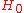was estimated to be between 50 and .

The value of the Hubble constant was the topic of a long and rather bitter controversy between Gérard de Vaucouleurs
Gérard de Vaucouleurs

who claimed the value was around 100 and Allan Sandage
Allan Sandage
Allan Rex Sandage was an American astronomer. He was Staff Member Emeritus with the Carnegie Observatories in Pasadena, California. He is best known for determining the first reasonably accurate value for the Hubble constant and the age of the universe.-Career:Sandage was one of the most...

who claimed the value was near 50. In 1996, a debate moderated by John Bahcall between Gustav Tammann
Gustav Tammann
Gustav Andreas Tammann was the director of the Astronomical Institute of the University of Basel, Switzerland, European Space Agency member of the Space Telescope Advisory Team, and Member of Council of the European Southern Observatory. His research interests include supernovae and the...

and Sidney van den Bergh
Sidney van den Bergh
Sidney Van den Bergh, OC, FRS is a retired Canadian astronomer.Born in the Netherlands, son of businessman and politician Sidney James van den Bergh and grandson of Unilever co-founder Samuel van den Bergh, he showed an interest in science from an early age, learning to read with books on astronomy...

was held in similar fashion to the earlier Shapley-Curtis debate over these two competing values.

This wide variance in estimates was partially resolved with the introduction of the ΛCDM model
Lambda-CDM model
ΛCDM or Lambda-CDM is an abbreviation for Lambda-Cold Dark Matter, which is also known as the cold dark matter model with dark energy...

of the universe in the late 1990s. With the ΛCDM model observations of high-redshift clusters at X-ray and microwave wavelengths using the Sunyaev-Zel'dovich effect
Sunyaev-Zel'dovich effect
The Sunyaev–Zel'dovich effect is the result of high energy electrons distorting the cosmic microwave background radiation through inverse Compton scattering, in which the low energy CMB photons receive energy boost during collision with the high energy cluster electrons...

, measurements of anisotropies in the cosmic microwave background radiation
In cosmology, cosmic microwave background radiation is thermal radiation filling the observable universe almost uniformly....

, and optical surveys all gave a value of around 70 for the constant.

### Using Hubble space telescope data

The Hubble Key Project (led by Dr. Wendy L. Freedman, Carnegie Observatories) used the Hubble space telescope
Hubble Space Telescope
The Hubble Space Telescope is a space telescope that was carried into orbit by a Space Shuttle in 1990 and remains in operation. A 2.4 meter aperture telescope in low Earth orbit, Hubble's four main instruments observe in the near ultraviolet, visible, and near infrared...

to establish the most precise optical determination in May 2001 of , consistent with a measurement ofbased upon Sunyaev-Zel'dovich effect
Sunyaev-Zel'dovich effect
The Sunyaev–Zel'dovich effect is the result of high energy electrons distorting the cosmic microwave background radiation through inverse Compton scattering, in which the low energy CMB photons receive energy boost during collision with the high energy cluster electrons...

observations of many galaxy cluster
Galaxy cluster
A galaxy cluster is a compact cluster of galaxies. Basic difference between a galaxy group and a galaxy cluster is that there are many more galaxies in a cluster than in a group. Also, galaxies in a cluster are more compact and have higher velocity dispersion. One of the key features of cluster is...

s having a similar accuracy.

### Using WMAP data

The most precise cosmic microwave background radiation
In cosmology, cosmic microwave background radiation is thermal radiation filling the observable universe almost uniformly....

determinations were , by WMAP in 2003, and , for measurements up to 2006. The five year release from WMAP in 2008 found using WMAP-only data and when data from other studies was incorporated, while the seven year release in 2010 found using WMAP-only data and when data from other studies was incorporated.

These values arise from fitting a combination of WMAP and other cosmological data to the simplest version of the ΛCDM model. If the data is fitted with more general versions,tends to be smaller and more uncertain: typically around although some models allow values near 63 (km/s)/Mpc.

### Using Chandra X-ray Observatory data

In August 2006, using NASA's Chandra X-ray Observatory
Chandra X-ray Observatory
The Chandra X-ray Observatory is a satellite launched on STS-93 by NASA on July 23, 1999. It was named in honor of Indian-American physicist Subrahmanyan Chandrasekhar who is known for determining the maximum mass for white dwarfs. "Chandra" also means "moon" or "luminous" in Sanskrit.Chandra...

, a team from NASA's Marshall Space Flight Center
Marshall Space Flight Center
The George C. Marshall Space Flight Center is the U.S. government's civilian rocketry and spacecraft propulsion research center. The largest center of NASA, MSFC's first mission was developing the Saturn launch vehicles for the Apollo moon program...

(MSFC) found the Hubble constant to be , with an uncertainty of about 15%.
The consistency of the measurements from all these methods lends support to both the measured value ofand the ΛCDM model
Lambda-CDM model
ΛCDM or Lambda-CDM is an abbreviation for Lambda-Cold Dark Matter, which is also known as the cold dark matter model with dark energy...

.

### Acceleration of the expansion

A value formeasured from standard candle observations of Type Ia supernova
Type Ia supernova
A Type Ia supernova is a sub-category of supernovae, which in turn are a sub-category of cataclysmic variable stars, that results from the violent explosion of a white dwarf star. A white dwarf is the remnant of a star that has completed its normal life cycle and has ceased nuclear fusion...

e, which was determined in 1998 to be negative, surprised many astronomers with the implication that the expansion of the universe is currently "accelerating" (although the Hubble factor is still decreasing with time, as mentioned above in the Interpretation section; see the articles on dark energy
Dark energy
In physical cosmology, astronomy and celestial mechanics, dark energy is a hypothetical form of energy that permeates all of space and tends to accelerate the expansion of the universe. Dark energy is the most accepted theory to explain recent observations that the universe appears to be expanding...

and the ΛCDM model).

## Derivation of the Hubble parameter

Friedmann equations
The Friedmann equations are a set of equations in physical cosmology that govern the expansion of space in homogeneous and isotropic models of the universe within the context of general relativity...

: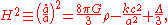whereis the Hubble parameter,is the scale factor
Scale factor (Universe)
The scale factor or cosmic scale factor parameter of the Friedmann equations is a function of time which represents the relative expansion of the universe. It is sometimes called the Robertson-Walker scale factor...

, G is the gravitational constant
Gravitational constant
The gravitational constant, denoted G, is an empirical physical constant involved in the calculation of the gravitational attraction between objects with mass. It appears in Newton's law of universal gravitation and in Einstein's theory of general relativity. It is also known as the universal...

,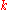is the normalised spatial curvature of the universe and equal to −1, 0, or +1, and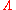is the cosmological constant
Cosmological constant
In physical cosmology, the cosmological constant was proposed by Albert Einstein as a modification of his original theory of general relativity to achieve a stationary universe...

.

### Matter-dominated universe (with a cosmological constant)

If the universe is matter-dominated
Matter-Dominated Era
The matter-dominated era was the epoch in the evolution of the Universe that began after the radiation-dominated era ended, when the Universe was about 70,000 years old. Although it was often said that we still live in the matter-dominated era, it is more correct to say that when the Universe was...

, then the mass density of the universe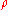can just be taken to include matter so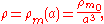where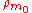is the density of matter today. We know for nonrelativistic particles that their mass density decreases proportional to the inverse volume of the universe so the equation above must be true. We can also define (see density parameter for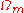)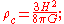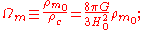so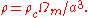Also, by definition,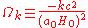and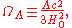where the subscript nought refers to the values today, and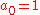. Substituting all of this in into the Friedman equation at the start of this section and replacingwith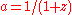gives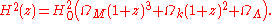### Matter- and dark energy-dominated universe

If the universe is both matter-dominated
Matter-Dominated Era
The matter-dominated era was the epoch in the evolution of the Universe that began after the radiation-dominated era ended, when the Universe was about 70,000 years old. Although it was often said that we still live in the matter-dominated era, it is more correct to say that when the Universe was...

and dark energy
Dark energy
In physical cosmology, astronomy and celestial mechanics, dark energy is a hypothetical form of energy that permeates all of space and tends to accelerate the expansion of the universe. Dark energy is the most accepted theory to explain recent observations that the universe appears to be expanding...

- dominated, then the above equation for the Hubble parameter will also be a function of the equation of state of dark energy
Equation of state (cosmology)
In cosmology, the equation of state of a perfect fluid is characterized by a dimensionless number \! w, equal to the ratio of its pressure \! p to its energy density \! \rho: \! w=p/\rho...

. So now: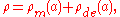where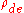is the mass density of the dark energy. By definition an equation of state in cosmology is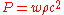, and if we substitute this into the fluid equation, which describes how the mass density of the universe evolves with time,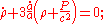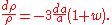If w is constant,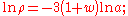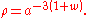Therefore for dark energy with a constant equation of state w,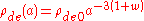. If we substitute this into the Friedman equation in a similar way as before, but this time set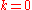which is assuming we live in a spatially flat universe, (see Shape of the Universe
Shape of the Universe
The shape of the universe is a matter of debate in physical cosmology over the local and global geometry of the universe which considers both curvature and topology, though, strictly speaking, it goes beyond both...

)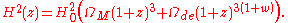If dark energy does not have a constant equation-of-state w, then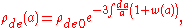and to solve this we must parametrize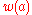, for example if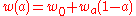, giving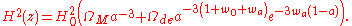### Hubble time

The Hubble constanthas units of inverse time, i.e.~ 2.3×10−18 s−1. “Hubble time” is defined as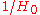. The value of Hubble time in the standard cosmological model
Lambda-CDM model
ΛCDM or Lambda-CDM is an abbreviation for Lambda-Cold Dark Matter, which is also known as the cold dark matter model with dark energy...

is 4.35×1017 s or 13.8 billion years. The phrase "expansion timescale" means "Hubble time".http://ybmessager.free.fr/docs/wiki-journey.html If the value ofwere to stay constant, a naive interpretation of the Hubble time is that it is the time taken for the universe to increase in size by a factor of e (because the solution of dx/dt = xis x =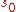exp(t), whereis the size of some feature at some arbitrary initial condition t = 0). However, over long periods of time the dynamics are complicated by general relativity
General relativity
General relativity or the general theory of relativity is the geometric theory of gravitation published by Albert Einstein in 1916. It is the current description of gravitation in modern physics...

, dark energy
Dark energy
In physical cosmology, astronomy and celestial mechanics, dark energy is a hypothetical form of energy that permeates all of space and tends to accelerate the expansion of the universe. Dark energy is the most accepted theory to explain recent observations that the universe appears to be expanding...

, inflation, etc., as explained above.

### Hubble length

The Hubble length or Hubble distance is a unit of distance in cosmology, defined as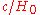—the speed of light multiplied by the Hubble time. It is equivalent to 4228 million parsecs or 13.8 billion light years. (The numerical value of the Hubble length in light years is, by definition, equal to that of the Hubble time in years.) The Hubble distance would be the distance at which galaxies are currently receding from us at the speed of light, as can be seen by substituting into the equation for Hubble's law, .

### Hubble volume

The Hubble volume is sometimes defined as a volume of the universe with a comoving
Comoving distance
In standard cosmology, comoving distance and proper distance are two closely related distance measures used by cosmologists to define distances between objects...

size of. The exact definition varies: it is sometimes defined as the volume of a sphere with radius, or alternatively, a cube of side. Some cosmologists even use the term Hubble volume to refer to the volume of the observable universe
Observable universe
In Big Bang cosmology, the observable universe consists of the galaxies and other matter that we can in principle observe from Earth in the present day, because light from those objects has had time to reach us since the beginning of the cosmological expansion...

, although this has a radius approximately three times larger.